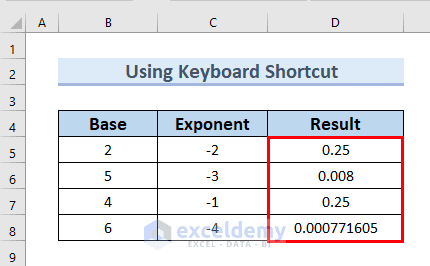# How to Use Negative Exponents in Excel (3 Easy Methods)Get FREE Advanced Excel Exercises with Solutions!

If you want to use negative exponents in Excel, you have come to the right place. Here, we will walk you through 3 easy and effective methods to do the task smoothly.

## How to Use Negative Exponents in Excel: 3 Easy Methods

In the following dataset, you can see the Base and Exponent columns. Here, you can easily notice that the Exponent column has negative exponents. Furthermore, using this dataset, we will go through 3 easy methods to use negative exponents in Excel. Here, we used Excel 365. You can use any available Excel version.### 1. Use of Keyboard Shortcut to Use Negative Exponents in Excel

In this method, we will use the keyboard shortcut SHIFT+6 to use negative exponents in Excel. Here, the SHIFT+6 keys will bring out the ^ symbol, which is used to type the power over a base number. We can use the negative exponent after the ^ symbol.

Steps:

• In the beginning, we will type the following formula in cell D5.
`=B5^C5`

Here, first, we typed =B5, and after that, we used the SHIFT+6 keys to bring out the ^ symbol. Then, we type C5.• After that, press ENTER.

Therefore, you can see the result in cell D5.

• Furthermore, we will drag down the formula with the Fill Handle tool.Therefore, you can see the complete Result column.Read More: How to Use Exponent Symbol in Excel

### 2. Using POWER Function in Excel

In this method, we will use the POWER function to use negative exponents in Excel. This is a quick and handy method to use negative exponent.

Steps:

First of all, we will type the following formula in cell D5.

`=POWER(B5,C5)`Formula Breakdown

• POWER(B5,C5) → The POWER function returns the outcome of a number raised to a power.
• B5 is the number.
• C5 → is the power.
• Outcome: 0.25
• Explanation: Here, 25 is the result of the base 2 raised to a negative exponent -2.
• After that, press ENTER.

At this point, you can see the result in cell D5.

• Furthermore, we will drag down the formula with the Fill Handle tool.As a result, you can see the complete Result column.Read More: How to Calculate Exponential in Excel

### 3. Employing EXP Function for Using Negative Exponents

Here, in the Base column, you can see the base is e (Euler’s Number). Further, we will find out the negative exponent of base e using the EXP function.

Steps:

• In the beginning, we will type the following formula in cell D5.
`=EXP(C5)`Formula Breakdown

• EXP(C5) → the EXP function returns e (Euler’s Number) raised to the power of number.
• C5 is the number
• Output: 0.135335283
• Then, press ENTER.

Therefore, you can see the result in cell D5.

• Furthermore, we will drag down the formula with the Fill Handle tool.Hence, you can see the complete Result column.Read More: How to Display Exponents in Excel

## How to Use Positive Exponents in Excel

Here, we will show you how you can use positive exponent in Excel. In the following dataset, you can in the Exponent column, the values of the exponents are positive. Then, we will use the POWER function to use positive exponents in Excel.Steps:

• In the first place, we will type the following formula in cell D5.
`=POWER(B5,C5)`Formula Breakdown

•  POWER(B5,C5) → The POWER function returns the outcome of a number raised to a power.
• B5 is the number.
• C5 → is the power.
• Outcome: 4
• Explanation: Here, 4 is the result of the base 2 raised to a positive exponent 2.
• After that, press ENTER.

At this point, you can see the result in cell D5.

• Furthermore, we will drag down the formula with the Fill Handle tool.As a result, you can see the complete Result column.## Practice Section

You can download the above Excel file to practice the explained method.## Conclusion

Here, we tried to show you 3 easy methods to use negative exponent in Excel. Thank you for reading this article, we hope this was helpful. If you have any queries or suggestions, please let us know in the comment section below.

## Related ArticlesAfia Kona

Hello, I am Afia Aziz Kona. I graduated in Naval Architecture and Marine Engineering from Bangladesh University of Engineering and Technology (BUET). I have an immense interest in technical writing and content development, therefore, I am working as a content developer at Exceldemy. In my spare time, I travel, watch movies, and cook different dishes.

We will be happy to hear your thoughtsAdvanced Excel Exercises with Solutions PDF